# 17 Results

View
Selected filters:
• Word Problems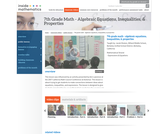قراءة النص المطبوع بخط رفيع
Rating

يدور هذا الدرس حول محاولة جعل الطلاب يقيمون روابط بين الأفكار عن المعادلات والمتباينات والمقادير الجبرية. علماً بأن الدرس مصمم ليمنح الطلاب فرص استخدام المفردات الرياضية لغرض الوصف والمناقشة والعمل مع سلاسل الرموز هذه. وتهدف الفكرة إلى بدء الطلاب في تحصيل المعلومة الشاملة بالنظر إلى كامل سلسلة الأعداد بدلاً من التفكير فقط في إجراءات أو خطوات فردية. ومن المأمول أن يبدأ الطلاب في رؤية سلاسل الرموز كأغراض رياضية لها مجموعتها الفريدة من الصفات الخاصة بها. (رياضيات الصف السابع)

نوع المادة:
Activity/Lab
Lecture
Lesson Plan
Teaching/Learning Strategy
Provider:
Noyce Foundation
Provider Set:
في قلب الرياضيات
المؤلف:
Disston, Jacob
11/30/2011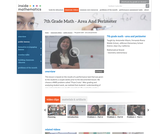قراءة النص المطبوع بخط رفيع
Rating

يستند هذا الدرس على نتائج مهمة الأداء الذي أدركنا منه أن فهم الطلاب للمساحة والمحيط كانت معظمها إجرائية. لذلك كان الغرض من هذا الدرس إعادة الانخراط لمعالجة المفاهيم الخاطئة لدى الطالب وتعميق فهم الطلاب للمساحة والمحيط. ان المعايير التي تم تناولها في هذا الدرس تنطوي على إيجاد محيط ومساحة الأشكال المختلفة، وإيجاد المحيط عند إعطاء مساحة ثابتة، واستخدام الصيغة في سياق عملي. وشملت التحديات التي تواجه طلابنا فك رموز اللغة في المشكلة واثبات طريقة تفكيرهم. (الصف 7 الرياضيات)

نوع المادة:
Activity/Lab
Lecture
Lesson Plan
Teaching/Learning Strategy
Provider:
Noyce Foundation
Provider Set:
في قلب الرياضيات
المؤلف:
Villarin, Antoinette
11/30/2011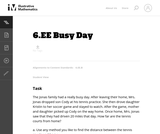Unrestricted Use
CC BY
Rating

This task provides a good entry point for students into representing quantities in contexts with variables and expressions and building equations that reflect the relationships presented in the context.

نوع المادة:
Activity/Lab
Provider:
Illustrative Mathematics
Provider Set:
Illustrative Mathematics
المؤلف:
Illustrative Mathematics
08/15/2012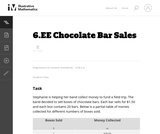Unrestricted Use
CC BY
Rating

In this task students use different representations to analyze the relationship between two quantities and to solve a real world problem. The situation presented provides a good opportunity to make connections between the information provided by tables, graphs and equations.

نوع المادة:
Activity/Lab
Provider:
Illustrative Mathematics
Provider Set:
Illustrative Mathematics
المؤلف:
Illustrative Mathematics
08/08/2012قراءة النص المطبوع بخط رفيع
Rating

The foundation of this lesson is constructing, communicating, and evaluating student-generated tables while making comparisons between three different financial plans. Students are given three different DVD rental plans and asked to analyze each one to see if they could determine when the 3 different DVD plans cost the same amount of money, if ever. (7th/8th Grade Math)

نوع المادة:
Activity/Lab
Lecture
Lesson Plan
Teaching/Learning Strategy
Provider:
Noyce Foundation
Provider Set:
في قلب الرياضيات
المؤلف:
Dimas, Cecilio
11/30/2011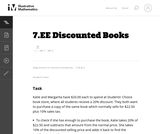Unrestricted Use
CC BY
Rating

This purpose of this task is to help students see two different ways to look at percentages both as a decrease and an increase of an original amount. In addition, students have to turn a verbal description of several operations into mathematical symbols.

نوع المادة:
Activity/Lab
Provider:
Illustrative Mathematics
Provider Set:
Illustrative Mathematics
المؤلف:
Illustrative Mathematics
05/01/2012Unrestricted Use
CC BY
Rating

In this task students are asked to write an equation to solve a real-world problem. There are two natural approaches to this task. In the first approach, students have to notice that even though there is one variable, namely the number of firefighters, it is used in two different places. In the other approach, students can find the total cost per firefighter and then write the equation.

نوع المادة:
Activity/Lab
Provider:
Illustrative Mathematics
Provider Set:
Illustrative Mathematics
المؤلف:
Illustrative Mathematics
05/01/2012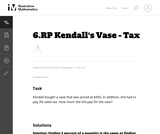Unrestricted Use
CC BY
Rating

This word problem requires students to compute sales tax.

نوع المادة:
Activity/Lab
Provider:
Illustrative Mathematics
Provider Set:
Illustrative Mathematics
المؤلف:
Illustrative Mathematics
05/01/2012قراءة النص المطبوع بخط رفيع
Rating

This lesson is a re-engagement lesson designed for learners to revisit a problem-solving task they have already experienced. Students will activate prior knowledge of graphical representations through the 'what's my rule' number talk; compare and contrast two different learners' interpretations of the growing pattern; use multiple representations to demonstrate how one of these learners would represent the numeric pattern; make connections between the different representations to more critically compare the two interpretations. (5th/6th Grade Math)

نوع المادة:
Activity/Lab
Lecture
Lesson Plan
Teaching/Learning Strategy
Provider:
Noyce Foundation
Provider Set:
في قلب الرياضيات
المؤلف:
Dickinson, Fran
11/30/2011Unrestricted Use
CC BY
Rating

This real world word problem requires multiple steps.

نوع المادة:
Activity/Lab
Provider:
Illustrative Mathematics
Provider Set:
Illustrative Mathematics
المؤلف:
Illustrative Mathematics
05/01/2012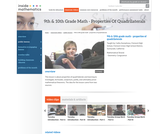قراءة النص المطبوع بخط رفيع
Rating

This lesson is about properties of quadrilaterals and learning to investigate, formulate, conjecture, justify, and ultimately prove mathematical theorems. Students will: Analyze characteristics and properties of two- and three-dimensional geometric shapes; develop mathematical arguments about geometric relationships; and apply appropriate techniques, tools, and formulas to determine measurements.Explore relationships among classes of two- and three-dimensional geometric objects, make and test conjectures about them, and solve problems involving them. Employ forms of mathematical reasoning and proof appropriate to the solution of the problem at hand, including deductive and inductive reasoning, making and testing conjectures, and using counter examples and indirect proof. Identify, formulate and confirm conjectures. Establish the validity of geometric conjectures using deduction, prove theorems, and critique arguments made by others. (9th/10th Grade Math)

نوع المادة:
Activity/Lab
Lecture
Lesson Plan
Teaching/Learning Strategy
Provider:
Noyce Foundation
Provider Set:
في قلب الرياضيات
المؤلف:
Humphreys, Cathy
11/30/2011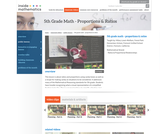قراءة النص المطبوع بخط رفيع
Rating

This lesson is about ratios and proportions using candy boxes as well as a recipe for making candy as situations to be considered. It addresses many Mathematical Reasoning standards and asks students to: Use models to understand fractions and to solve ratio problems; think about a ratio as part/part model and to think about the pattern growing in equal groups or a unit composed of the sum of the parts; find a scale factor and apply it to a ratio. (5th Grade Math)

نوع المادة:
Activity/Lab
Lecture
Lesson Plan
Teaching/Learning Strategy
Provider:
Noyce Foundation
Provider Set:
في قلب الرياضيات
المؤلف:
Lewis-Wolfsen, Hillary
11/30/2011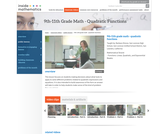قراءة النص المطبوع بخط رفيع
Rating

This lesson focuses on students making decisions about what tools to apply to solve different problems related to quadratic expressions and equations. It is also intended to build awareness of the form an answer will take in order to help students make sense of the kind of problem they are solving. (9th/10th/11th Grade Math)

نوع المادة:
Activity/Lab
Lecture
Lesson Plan
Teaching/Learning Strategy
Provider:
Noyce Foundation
Provider Set:
في قلب الرياضيات
المؤلف:
Shreve, Barbara
11/30/2011Unrestricted Use
CC BY
Rating

There are a couple of ways to solve this real world word problem.

نوع المادة:
Activity/Lab
Provider:
Illustrative Mathematics
Provider Set:
Illustrative Mathematics
المؤلف:
Illustrative Mathematics
05/01/2012Unrestricted Use
CC BY
Rating

The purpose of this task is to present students with a context that can naturally be represented with an inequality and to explore the relationship between the context and the mathematical representation of that context; thus, this is an intended as an instructional task.

نوع المادة:
Activity/Lab
Provider:
Illustrative Mathematics
Provider Set:
Illustrative Mathematics
المؤلف:
Illustrative Mathematics
08/10/2012Unrestricted Use
CC BY
Rating

This is a multi-step problem since it requires more than two steps no matter how it is solved. The problem is not scaffolded for the student, but each step is straightforward and should follow from the previous with a careful reading of the problem.

نوع المادة:
Activity/Lab
Provider:
Illustrative Mathematics
Provider Set:
Illustrative Mathematics
المؤلف:
Illustrative Mathematics
05/01/2012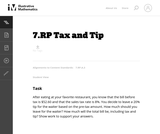Unrestricted Use
CC BY
Rating

This real world word problem about calculating a tip in a restaurant requires multiple steps.

نوع المادة:
Activity/Lab
Provider:
Illustrative Mathematics
Provider Set:
Illustrative Mathematics
المؤلف:
Illustrative Mathematics